Courses

# Short & Long Answer Question(Part-1) - Equilibrium Class 11 Notes | EduRev

## JEE : Short & Long Answer Question(Part-1) - Equilibrium Class 11 Notes | EduRev

The document Short & Long Answer Question(Part-1) - Equilibrium Class 11 Notes | EduRev is a part of the JEE Course Chemistry Class 11.
All you need of JEE at this link: JEE

Q.1 A crystal of common salt of given mass is kept in aqueous solution. After 12 hours, its mass remains the same. Is the crystal in equilibrium with the solution?

Answer: Yes, as the mass of the crystal remains the same, this means that the solution is saturated. Therefore, there is a physical equilibrium between the crystal of the common salt and its saturated solution.
Q.2 Why is there a fizz when a soda water bottle is opened?

Answer: In soda water bottle, CO2(g) is dissolved in water under high pressure since under normal pressure the solubility of the gas is small. Now, when the bottle is opened, the pressure of the gas inside the bottle decreases. As a result, the solubility of the gas decreases. Therefore, the dissolved gas escapes from the bottle and there is a fiz.
Q.3 Can equilibrium be achieved between water and its vapours in an open vessel? Explain your answer and say what happens eventually.

Answer: No; equilibrium cannot be achieved between water and its vapours in an open vessel. Since water vapous are escaping out of the container, to take their place more of liquid water changes to vapours. This process continues till the entire water has changed to the vapours.
Q.4 From the values of the equilibrium constants, indicate in which case, does the reaction go farthest to completion: K1=10−10,K2=1010,K3=105.

Answer: High value of equilibrium constant (K) means that the molar concentration of the products is quite large. In other words, the reaction has proceeded to a large extent in the forward direction before attaining the equilibrium. Thus, for K2=1010,  the forward reaction has proceeded maximum before attaining the equilibrium.
Q.5 The value of the equilibrium constant is less than zero. What does it indicate?

Answer: This indicates that the forward reaction has proceeded only to a small extent before the equilibrium is attained.
Q.6 Would you expect equilibrium constant for the reaction I2(g)⇌2I(g) to increase or decrease as temperature increases. Assign reason.

Answer: In the forward reaction, energy is needed to bring about the dissociation of I2(g) molecules. This means that the forward reaction is endothermic in nature. The increase in temperature will favour the forward reaction. Therefore, the equilibrium constant will increase with rise in temperature.
Q.7 Following equilibrium is set up when SCN  ion is added to Fe3+ in aqueous solution :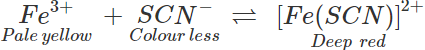When silver nitrate is added to the solution, AgSCN gets precipitate. What will happen to the equilibrium?

Answer: Equilibrium gets displaced to the left because with removal SCN ions from the equilibrium mixture, more of the deep red complex (product) will decompose into the reactants. Thus, the colour of the solution will slowly change from deep red to pale yellow.
Q.8 Following reaction occurs in a Blast Furnace: Fe2O3(s)+3CO(g)⇌2Fe(l)+3CO2(g) Use Le Chatelier's principle to predict the direction of reaction when equilibrium mixture is disturbed by (a) adding Fe2O3  (b) removing CO2  (c) removing CO.

Answer: (a) On adding Fe2O3(s), the equilibrium will remain unaffected. (b) By removing CO2(g), the equilibrium will be shifted in the forward direction (c) By removing CO(g), the equilibrium will be shifted in the backward direction.
Q.9 Acetic acid is highly soluble in water but still a weak electrolyte. Why?

Answer: The strength of the electrolyte does not depend upon its amount present in solution but on its ionisation in solution. Since acetic acid is a weak acid (organic acid), it is ionised only to small extent. Therefore, it is a weak electrolyte.
Q.10 How will you account for the following: (a) Clothes dry quicker on a windy day (b) We sweat more on a humid day?

Answer: (a) When wet clothes are spread on a stand, the water evaporates and the surrounding air tends to get saturated thus hampering the process of drying. On a windy day when breeze blows, the nearby wet air is replaced by dry air which helps the process of evaporation further. Thus, clothes dry quicker when there is a breeze. (b) The explanation is the same as given above. On a humid day, the air is already saturated with water vapours. This means that the water that comes out of the pores of the body as sweat does not vaporise. This will result in greater sweating on a humid day.
Q.11 The solubility of CO2 in water decreases with increase in temperature. Explain.

Answer: The dissolution of CO2 gas in water is exothermic in nature because the gas contracts in volume. Thus, on increasing the temperature, the speed of the endothermic or reverse process will increase according to Le Chatelier's principle. Therefore, the solubility of CO2  in water will decrease with the increase in temperature.
Q.12 Will ionic product of water increase or decrease if temperature is increased?

Answer: The ionic product of water (H2O) will increase upon increasing the temperature because more of ions will be formed.
Q.13 In a chemical reaction under equilibrium, there is no change in moler concentration of products and reactants. Does the reaction stop?

Answer: No, it does not stop. The equilibrium is of dynamic nature.
Q.14 Out of water and silver nitrate solution, in which case silver chloride will dissolve more?

Answer: Silver chloride will dissolve more in water than in silver nitrate solution because the latter has Ag+ ions as the common ions. Therefore, the solubility of silver chloride will be suppressed in silver nitrate solution.
Q.15 Magnesium is not precipitated from a solution of its salt by NH4OH in the presence of NH4Cl. Explain.

Answer: Mg2+ ions present in its salt solution are to be precipitated as Mg(OH)2 on adding NH4OH. But the presence of NH4Cl will suppress the ionisation of NH4OH  due to common ion effect. As a result, the ionic product of Mg2+ and OH ions will remain less than the Ksp value of Mg(OH)2. It will not be, therefore, precipitated.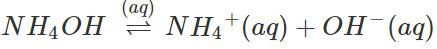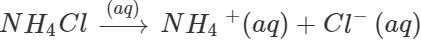Q.16 SO3 is an acidic oxide while Na2O  is basic in nature. Support the same with the help of examples.

Answer: (i) SO3 is an acidic oxide because it dissolves in water to form H2SO4  (acid) and also forms a salt with NaOH (base). SO3+H2O→H2SO4 SO3+2NaOH→Na2SO4+H2O (ii) Na2O is basic oxide because it dissolves in water to form NaOH(base) and also forms a salt with HCl (acid) Na2O+H2O→2NaOH Na2O+2HCl→2NaCl+H2O
Q.17 Zn(OH)2 is not precipitated when NH4OH  is added to a zinc salt solution containing some ammonium chloride. Explain.

Answer: Zn(OH)2 can precipitated if the product of the ionic concentration of the ions furnished by the salt in aqueous solution exceeds the Ksp value of the hydroxide. Addition of NH4Cl to the aqueous solution of the zinc salt in which NH4OH has been added as the precipitating reagent will suppress the OH  ions concentration in solution due to common ion effect (NH4+ ions being the common ions). This means that the concentration of the OH ions in solution will be sufficiently suppressed or decreased and the product of ionic concentration will be less than the Ksp  value of Zn(OH)2 and it will not be, therefore, precipitated.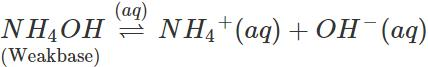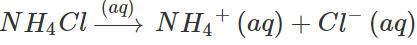Q.18 Will pH of water be same or different at 4C and 25C? Explain.

Answer: pH of water will be different at these temperatures. Rather pH of 4C will be more than pH at 25C. Actually, for water (neutral): [H+]×[OH]=Kw=10−14 at 25C Similarly, pH×pOH=pKw=14 at 25∘C or pH=pOH=7 (∵ Water is neutral andpH=pOH) On increasing the temperature, dissociation of water increases and so is the value of Kw. This means that at lower temperature (say 4C), the value of Kw will decrease or pKw  will be more than 14. This also means that at lower temperature, the value of pH (orpOH ) will be more than 7.
Q.19 Why gas fizzes out when soda water bottle is opened?

Answer: The amount of the gas dissolved is very high due to high pressure. On opening the bottle, the pressure tends to decrease to atmospheric pressure. So the solubility decreases, i.e., the dissolved gas escapes out.
Q.20 Reaction between acetic acid and ethyl alcohol attains a state of equilibrium in an open vessel but decomposition of CaCO3 does not. Why?

Answer: Acetic acid and ethyl alcohol are liquids and their products, ethyl acetate and water, are also liquids but one of the products of decomposition of CaCO3 is gaseous (CO2) which escapes out and the reverse reaction cannot occur.
Q.21 At equilibrium, the mass of each of the reactants and products remains constant. Does it mean that the reaction has stopped? Explain.

Answer: No, the reaction does not stop. It continues to take place in the forward as well as backward directions but at equal speeds.
Q.22 If concentrations are expressed in moles L−1 and pressures in atmospheres, what is the ratio of Kp to Kfor the reaction :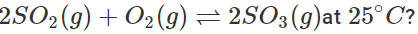Δng=np−nr=−1. Hence, Kp=Kc(RT)−1 or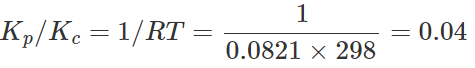Q.23 The value of equilibrium constant depends on what?

Answer: The value of equilibrium constant depends upon (i) nature of the reaction (ii) temperature.
Q.24 The equilibrium constant for the reactions (i) N2+O2⇌2NO and (ii) 2NO+O2⇌2NO2 are K1 and K2 respectively, then what will be the equilibrium constant for the reaction N2+2O2⇌2NO2?

Answer: Adding eqns. (i) and (ii), we get the required equation. Thus, (i) and (ii) are the two steps of the required equation. Hence, for the required reaction, K=K1×K2.
Q.25  For the reaction N2(g)+3H2(g)⇌2NH3(g),at 400K,Kp=41. Find the value of Kp for each of the following reactions at the same temperature: (i) 2NH3(g)⇌N2(g)+3H2(g)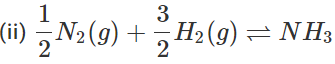(iii)2N2(g)+6H2(g)⇌4NH3(g)

Answer:(i) It is the reverse of the given reaction. Hence, Kp= 1/41 (ii) It is obtained by dividing the given equation by 2. Hence, Kp=√41 (iii) It is obtained by multiplying the given equation by 2. Hence, Kp=(41)2.
Q.26 For an exothermic reaction, what happens to the equilibrium constant if temperature is increased?

Answer: K=kf/kb. In exothermic reaction, with increase of temperature, kb increases much more than kf.  Hence, K decreases.
Q.27 The equilibrium constant of a reaction is 2×10−3 at 25°C and 2×10−2 at 50°C. Is the reaction exothermic or endothermic?

Answer: As equilibrium constant has increased with temperature, the reaction is endothermic (in the forward direction).
Q.28 The equilibrium H2O(l)⇌H2O(υ) is attained in a closed container at 40°C. The aqueous tension of water at 40° C is 23 mm. What is Kp for the said equilibrium?

Q.29 What does the equilibrium constant K < 1 indicate?

Answer: The reaction does not proceed much in the forward direction.
Q.30 What qualitative information can you obtain from the magnitude of equilibrium constant?

Answer: (i) Large value of equilibrium constant (>103) shows that forward reaction is favoured, i,e., concentration of products is much larger than that of the reactants at equilibrium. (ii) Intermediate value of K(10−3 to 103) shows that the concentration of the reactants and products are comparable. (iii) Low value of K(<10−3) shows that backward reaction is favoured, i.e., concentration of reactants is much larger than that of the products.
Q.31 In which one of the following reactions, the yield of the product will be maximum? 2A+B⇌C,K=10−5;C+2D⇌E,K=105;D+3B⇌F,K=103

Answer: Higher the value of equilibrium constant K, greater is the yield of products. Hence, yield of E will be maximum.

Q.32 For the reaction: H2+I2⇌2HI, if initially 25 mL of Hand 20 mL of I2 are present in a container and at equilibrium, 30 mL of HI is formed, then calculate equilibrium constant.

Answer: H2 + I2  ⇌    2HI Initial:  25mL 20mL  0 At eqm. :25−x 20−x 2x But 2x=30mL (Given), i.e., x=15 ∴ At equilibrium, H2=25−15=10mL,I2=20−15=5mL and HI=30mL. As equal volumes contain equal number of moles, therefore, volumes can be used in place of moles. Hence,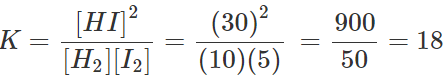Q.33 What two changes on the equilibrium,
N2(g)+3H2(g)⇌2NH3(g),ΔH=−92.4kJ. can keep its state undisturbed?

Answer: Increase of temperature along with suitable increase of pressure or increase of pressure along with suitable increase of temperature.
Q.34 The following reaction has attained equilibrium CO(g)+2H2(g)⇌CH3OH(g),ΔH=−92.0kJmol−1 What will happen if (i) volume of the reaction vessel is suddenly reduced to half? (ii) the partial pressure of hydrogen is suddenly doubled? (iii) an inert gas is added to the system?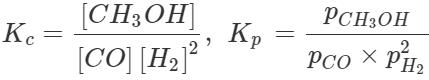(i) When volume of the vessel is reduced to half, the concentration of each reactant or product becomes double. Thus,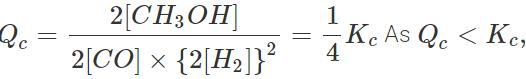equilibrium will shift in the forward direction, producing more of  CH3OH to make Qc=Kc. (ii)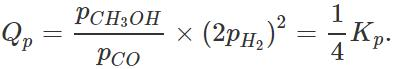Again, Qp<Kp,equilibrium will shift in the forward direction to make Qp=Kp. (iii) As volume remains constant, molar concentrations will not change. Hence, there is no effect on the state of equilibrium.
Q.35 The following system is in equilibrium : SO2Cl2+Heat⇌SO2+Cl2 What will happen to the temperature of the system if some Cl2 is added into it at constant volume? Give reason.

Answer: Temperature of the system will increase because on adding Cl2, equilibrium will shift in the backward direction producing more heat.
Q.36 A reaction A(g)+B(g)⇌2C(g) is in equilibrium at a certain temperature. Can we increase the amount of products by (i) adding catalyst (ii) increasing pressure?

Answer: (i) No, because catalyst does not disturb the state of equilibrium. (ii) No, because np=nr
Q.37 Why does ice melt slowly at higher altitudes?

Answer: Melting of ice is endothermic process accompanied by decrease of volume. At altitudes, pressure as well as temperature is low. In the equilibrium, Ice ⇌ Water, both the factors favour backward process. Hence, the melting is slow.

Q.38 2N2O(g)+O2(g)⇌4NO(g);ΔH>0 What will be the effect on equilibrium when (i) Volume of the vessel increases? (ii) Temperature decreases?

Answer: (i) For the given reaction,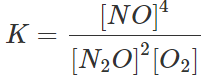When volume of the vessel increases, number of moles per unit volume (i.e. molar concentration) of each reactant and product decreases. As there are 4 concentration terms in the numerator but 3 concentration terms in the denominator, to keep K constant, the decrease in [N2O] and [O2] should be more, i.e., equilibrium will shift in the forward direction. Alternatively, increase in volume of the vessel means decrease in pressure. As forward reaction is accompanied by increase in the number of moles (i.e. increase of pressure), decrease in pressure will favour forward reaction (according to Le Chatelier's principle). (ii) As ΔHis +ve, i.e., reaction is endothermic, decrease of temperature will favour the direction in which heat is absorbed, i.e., backward direction.
Q.39 Some processes are given below. What happens to the process if it is subjected to a change given in the brackets? (i)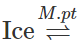Water (Pressureisincreased) (ii) Dissolution of NaOH in water (Temperature is increased) (iii)N2(g)+O2(g)⇌2NO(g)−180.7kJ (Pressure is increased and temperature is decreased).

Answer: (i) Equilibrium will shift in the forward direction, i.e., more of ice will melt. (ii) Solubility will decrease because it is an exothermic process. (iii) Pressure has no effect. Decrease of temperature will shift the equilibrium in the backward direction.
Q.40 How does the degree of ionization of a weak electrolyte vary with concentration? Give exact relationship. What is this law called?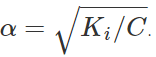It is called Ostwald's dilution law (Ki is ionization constant and C is molar concentration of the electrolyte).
Q.41 Write down the conjugate acid and conjugate base of (i) H2O (ii) HSO4 (iii) NH3 (iv) HS

Answer: (i) H3O+,OH(ii) H2SO4,SO2−4(iii) NH+4,NH−2(iv) H2S,S2− Note. To write the conjugate acid of the given species, add H+ ion. To write the conjugate base of the given species, subtract H+ ion.
Q.42 Out of CH3COOand OH which is stronger base and why?

Answer: OH ions can combine with H+ ions more readily than CH3COO ions can do. Hence, OH is a stronger base. Alternatively, the conjugate acid of CH3COO and OH are CH3COOH and H2O. As CH3COOH is stronger acid than H2O, the conjugate base of CH3COOH, viz.,CH3COO will be a weaker base.
Q.43 Why is ammonia termed as a base though it does not contain OH ions?

Answer: NH3 is termed as a base on the basis of Lewis concept because it can donate a lone pair of electrons.
Q.44 Arrange the given species in order of their increasing basic character : H2O,OH,CH3OH,CH3O

Answer: Applying the concept that a strong acid has a weak conjugate base, order of basic character is : H2O<CH3OH<OH<CH3O
Q.45 Between Na+ and Ag+ which is stronger Lewis acid and why?
Ag+ This is because Ag+ has a pseudo noble gas configuration which makes it more polarizing.
Q.46 How is the degree of dissociation of a weak base related to its molarity?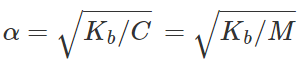where M = molarity.
Q.47 lonization constants Ka for formic acid and acetic acid are 17⋅7×10−5 and 1⋅77×10−5. Which acid is stronger and how many times the other if equimolar concentrations of the two are taken?

Answer: Ka for HCOOH>Ka for CH3COOH. Hence, formic acid is stronger.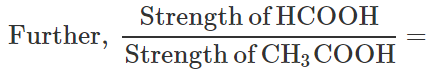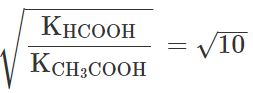= 3⋅16 times.
Q.48 What is the effect of temperature on ionic product of water and why?

Answer: Ionic product of water (Kw) increases with increase of temperature because Kw=[H3O+][OH] and dissociation of H2O to give H3O+ and OHincreases with increase of temperature.
Q.49 What happens to the ionic product of water if some acid is added into water?

Q.50 What are pH and pOH values of a neutral solution at a temperature at which Kw=10−13?

Answer: pKw=pH+pOH. But pKw=13. Also, for neutral solution, pH=pOH. Hence, pH=pOH=6.5.

Offer running on EduRev: Apply code STAYHOME200 to get INR 200 off on our premium plan EduRev Infinity!

## Chemistry Class 11

136 videos|245 docs|180 tests

,

,

,

,

,

,

,

,

,

,

,

,

,

,

,

,

,

,

,

,

,

;